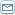# Profit From Forex Trading With Moving Averages

 By: Ian ArmstrongWhen you forecast Forex prices for the future, you use moving averages as a charting tools that will help in your predictions. There are several different kinds of moving averages.Simple Moving Averages You calculate a simple moving average (also known as SMA) by dividing the total of the past chosen period closing prices by the number of periods. Moving averages work on delay because you use price information from past periods to try and determine events for subsequent ones. It's important to note here that past trends do not guarantee future trends, however. When the periods you use to calculate your moving average are smaller lengths of time, your chart will be "choppier" versus if you use bigger periods of time; with this, the chart will be less uneven. However, if you use larger periods of time, your moving averages will also be less reactive to price changes. This is especially true with simple moving averages, because contributions to moving averages are the same for all individual periods.One moving average is quite useless as a tool. If you want to find the price trends in the Forex market, you'll need to plot a series of moving averages instead.Exponential Moving Averages (EMA)Although simple moving averages are good tools to quickly establish the Forex market trends, they can be very susceptible to fluctuations and also rely on older as well as newer prices; this makes them less accurate. When you do a technical analysis, you'll need to base forecasts on the most recent prices you have available to you. This means that you'll need to base your forecast on what traders in the market are doing right now, not on what they were doing yesterday, last week or last month.Exponential moving averages weight recent currency quotes to greater emphasis earlier currency quotes. For example, if the daily closing prices for the pair GBP/USD are:Day11.9722Day21.9727(1.9650)Day31.9737Day41.9742Day51.9747This means 1.9735 is the simple moving average with a five-period one-day chart as the example. This is higher than the price on the first day, and suggests that the pair GBP/USD will be going up. If on day two this figure is 1.9650, this may indicate an interest rate change by the Bank of England. The simple moving average here would be 1.9720, which indicates a downward trend for the currency pair GBP/USD. (However, the price then increased consistently from day two through day five.)What you need to do, then, is to use exponential moving averages that place more emphasis on recent prices. The exponential moving average, in other words, gives more emphasis to what the market is doing now instead of yesterday, the day before or last month.In the example just given above, day two's closing prices are at 1.9650, which means that the exponential moving average would have a weighting factor of 0.1, while 1.9726 would have a weighting factor of 0.2. (More recent prices are given higher weighting factors.)You don't need to do actual exponential moving averages calculations, because your charting software can do this once you plug the numbers in.